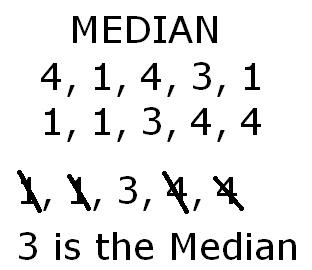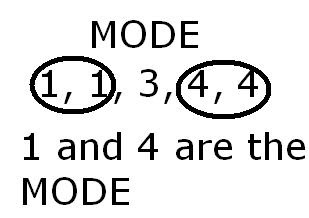### Maeddah's Measure of Central Tendency

Monday, September 29, 2008
Mean

- Arrange data in ascending order.(1, 1, 3, 4, 4)
- Add the set of data together. (1+1+3+4+4=13)
- Take the sum of data, and divide it by the numbers of data. (13/5=2.6)Median

- Arrange data in ascending order. (1, 1, 3, 4, 4)
- Cross out each number on the right and left until one number is left. (1, 1, 3, 4, 4)
- 3 is the middle number out of the data.
(If the numbers of data equal up to an equal number, you must take the last 2, add them together, and divide the sum by 2. 1, 1, 3, 4, 4, 5. 3+4= 7/2=3.5Mode

- Arrange data in ascending order. (1, 1, 3, 4, 4)
- Found the most occuring number/S. (1, 1, 3, 4, 4)
- 1 and 4 are the mode.
(There doesn't always have to be a Mode. There CAN be more than ONE Mode.)- Arrange data in ascending order (1, 1, 3, 4, 4)
- Look for the greatest piece of data (1, 1, 3, 4, 4)
- Look for the smallest piece of data (1, 1, 3, 4, 4)
- Subtract the greatest piece of data with the smallest piece of data (4-3=1)Heres a video about M,M,M!